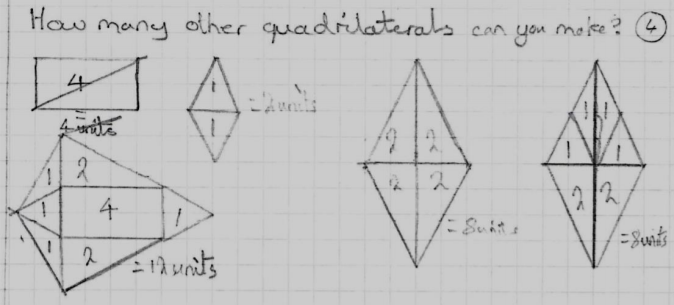#### You may also likeFour rods, two of length a and two of length b, are linked to form a kite. The linkage is moveable so that the angles change. What is the maximum area of the kite?### Making Rectangles, Making Squares

How many differently shaped rectangles can you build using these equilateral and isosceles triangles? Can you make a square?This gives a short summary of the properties and theorems of cyclic quadrilaterals and links to some practical examples to be found elsewhere on the site.

# An Equilateral Triangular Problem

##### Age 11 to 14 Challenge Level:

Mahdi from Mahatma Gandhi International School in India, Alyssa from NGHS in the UK and Julia from Tanglin Trust School in Singapore found all of the angles. This is Mahdi's work: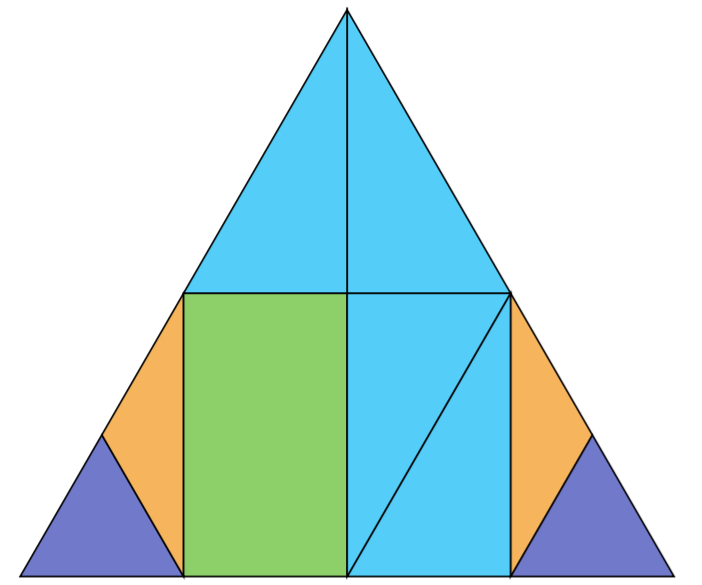Shapes with same colours are congruent. We can easily note this because all their sides are equal. We can also observe the following:

• We are given that the largest triangle is equilateral
• We also know that the purple triangles are equilateral (each side is $\frac14$ of the [side of the largest triangle]
• The blue triangles are right-angled triangles
• The orange triangles are isosceles triangles
• the green quadrilateral is a trctangle with all angles $90^\circ$

We can complete the angles using the above.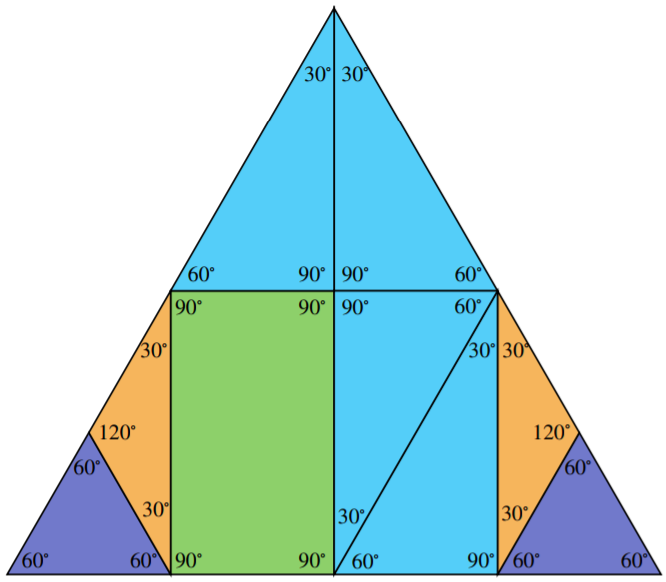Mahdi and Oscar, Dominic, Olivia, Jodie, Harriet, Rakesh and Mo from Westdene Primary School in the UK worked out the areas of the little shapes, where the area of the smallest equilateral triangle is 1 unit. This is Osacar and Dominic's diagram. They wrote: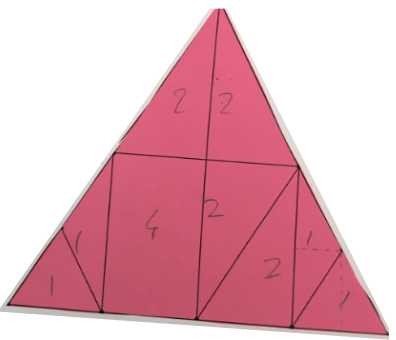We knew that the smallest equilateral triangle is worth one unit. We later knew that the small isosceles triangle and small equilateral triangle when halved had the same area in each half. Therefore the small isosceles triangle was equal in area to the small equilateral triangle.

Rakesh, Mo, Olivia, Jodie and Harriet described the same thing in a different way. This is Rakesh and Mo's work, including their method for the rectangle and the right-angled triangle:

[The areas of the] smallest equilateral triangles and the isosceles [triangles] are equal. You can prove this by placing the two equilateral triangles side by side and the two isosceles also side by side to make two identical rhombuses.

If you place the two smallest equilateral triangles on the width of the rectangle (bases along top and bottom) and the isosceles on the length (bases along left and right) you will find the rectangle to have an area of 4 units.

If you place the smallest equilateral [triangle] and the isosceles [triangle] on the right angled triangles you will find that the right angled triangles are 2 units.

The others used a different method for the rectangle. Olivia, Jodie and Harriet wrote:

A rectangle must be four units because it is double the right angle triangle.

Kevin from Malvern College Hong Kong, Josh and Amara from Westdene Primary School and Julia used a different method to find the areas of the shapes. Julia wrote:

The triangle can be separated in 16 one unit triangles.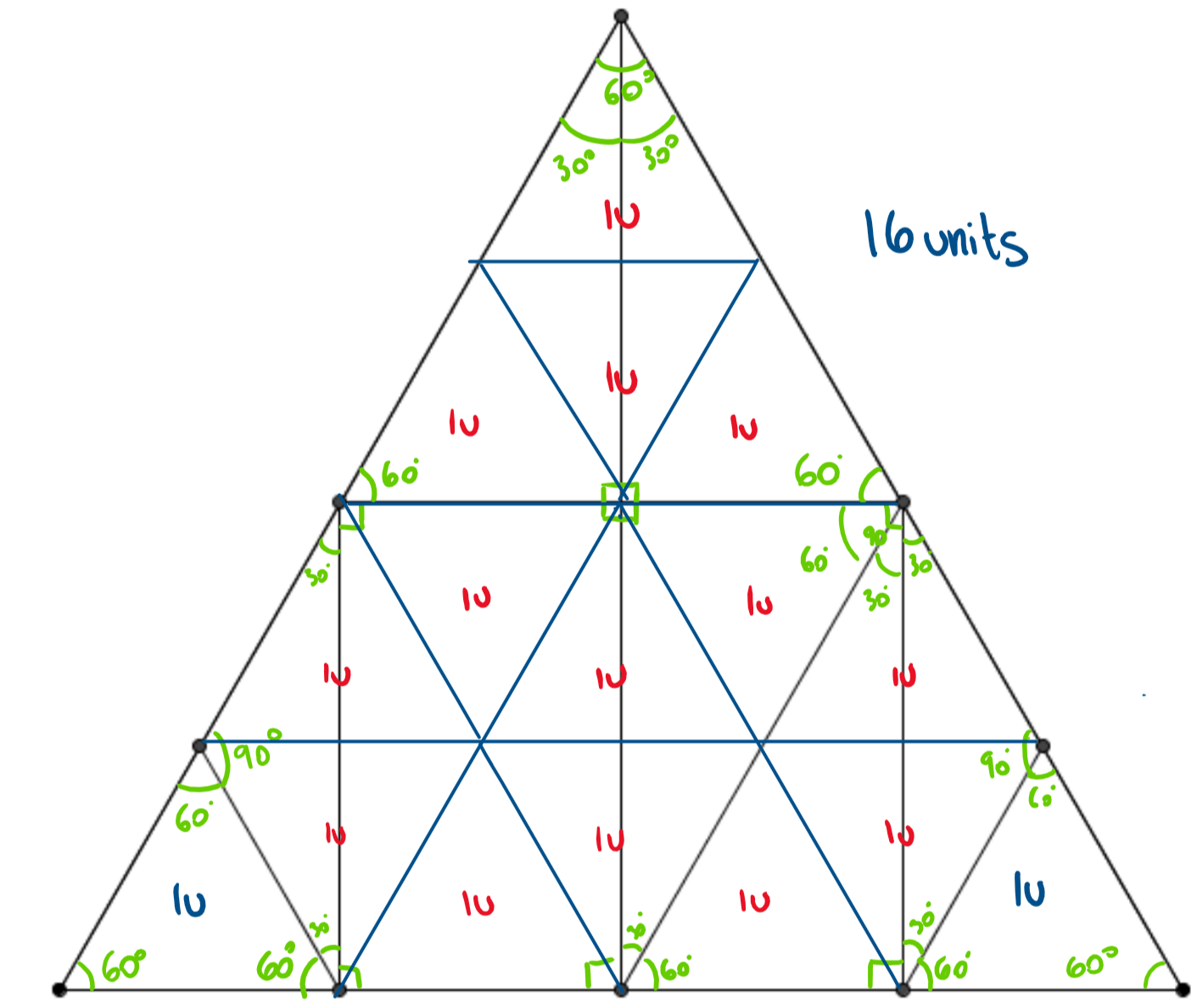Book and Priya from Bangkok Patana School in Thailand were able to find the angles in each of the cut out shapes and they were able to make 15 different trapezia.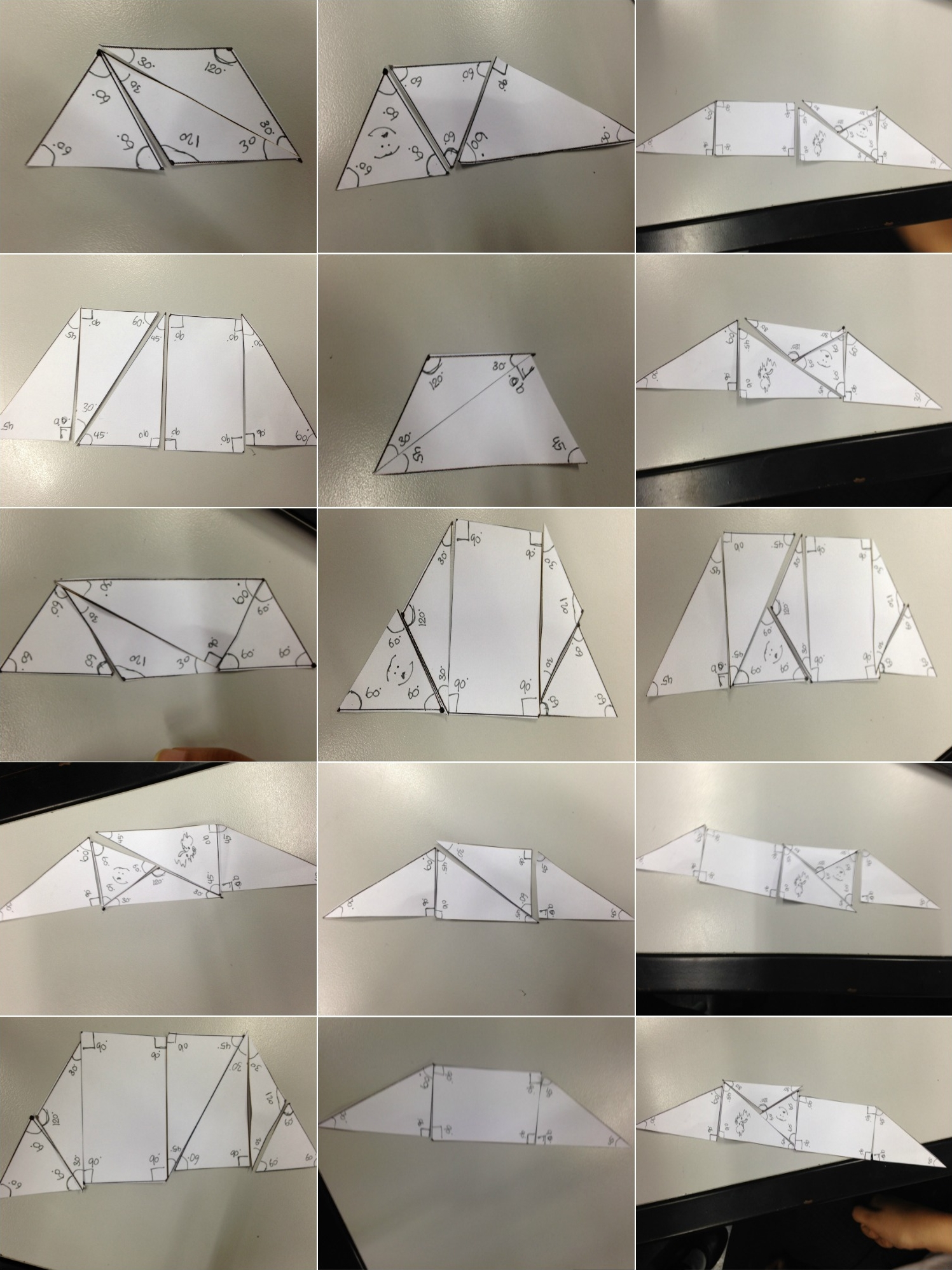Ethan from Meopham Community Academy in the UK and Kevin both found more different trapeziums, parallelograms, rectanges and other quadrilaterals. This is Kevin's work. Notice that Kevin's trapeziums are almost all different to Book and Priya's trapeziums.These are Ethan's trapeziums and parallelograms.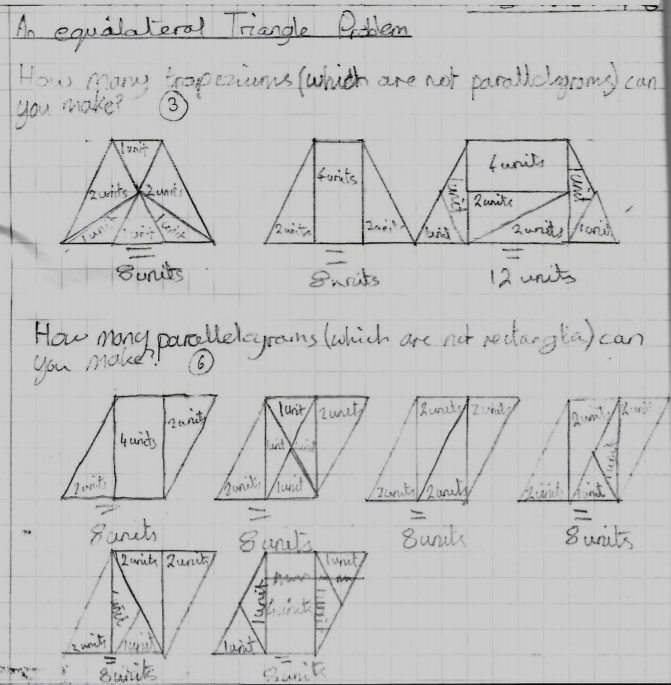Ethan found the same rectangles as Kevin, plus these: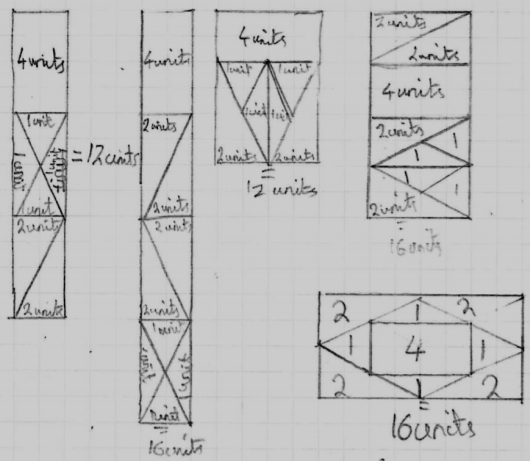Mahdi considered different ways to make rectangles congruent to the little rectangle to try and make a list of all of the possible rectangles. Some of Ethan's are missing from the list, but it is a very good systematic approach. Click here to see Mahdi's working and rectangles.

And Ethan found some other quadrilaterals that are different to any of Kevin's quadrilaterals: